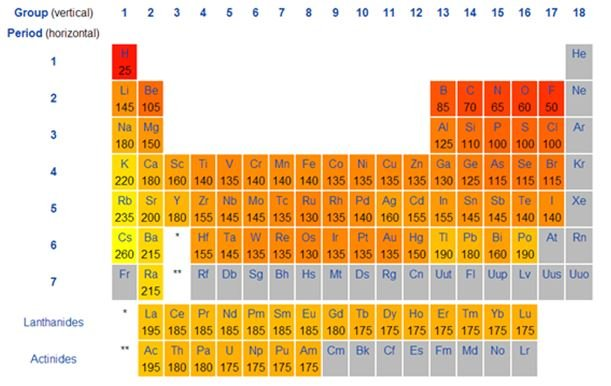It is the distance from the center of the nucleus to the point up to which the density of the electron cloud is maximum.

1. A. Size of the atom is an order of 1.2 Angstroms or 1.2 x 10-10m, which is too small to measure it.
2. B. Electron cloud around the atom has no well-defined boundary. Which also affects the precision of the measurement? However, it is possible to measure the radius or size of an atom by knowing the distance between atoms in combined state.
• Depending on the nature of bonding between atoms Atomic Radius is of three types:
3. 3. Van der Waals Radius
• Covalent radius is a general term used in case of Non-metals; it is one half of the distance between the nuclei of two atoms, held together by a covalent bond in a homo diatomic molecule.
• For example the inter-nuclear distance between chlorine atoms in chlorine molecule is 198pm.Therefore the radius of chlorine atom is half of this distance, i.e.99pm.where pm stands for Pico meter.• For metals we use the word metallic radius, Metallic radius is the half of the inter-nuclear distance between centers of the nuclei of two adjacent metal atoms in a metallic crystal.
• For example the distance between two adjacent copper atoms in solid copper is 256 pm.Hence, the metallic radius of copper atom is 128 pm.
• Covalent and metallic radius is measured by using X-Ray spectroscopy or other spectroscopy methods.
• Generally atomic radius decreases across the period and increases down the group.
• The decrease in the atomic radius across the period is due to decrease in effective nuclear charge with increase in atomic number.
• Down the group atomic number increases with increase in atomic number. As we move down the group a new shell is added, which means the distance between nucleus and valence shell increases down the group. Hence, when we move down the group atomic radius increases.

### Solved Example:

#### Ques: Which of the following metal has maximum metallic radius?.

A. Cesium
B. Potassium
C. Lithium
D. Sodium
Answer: A - Solution: When we move down the group metallic radius increases, the above elements are belongs to first group. Hencecesium metal has maximum metallic radius.